# Remainders

It is given a set of numbers { 170; 244; 299; 333; 351; 391; 423; 644 }. Divide this numbers by number 66 and determine set of remainders. As result write sum of this remainders.

Correct result:

s =  281

#### Solution: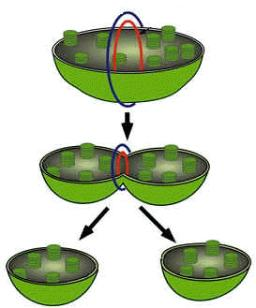We would be pleased if you find an error in the word problem, spelling mistakes, or inaccuracies and send it to us. Thank you!Tips to related online calculators
Do you want to perform natural numbers division - find the quotient and remainder?

## Next similar math problems:

• DivisibilityIs the number 761082 exactly divisible by 9? (the result is the integer and/or remainder is zero)
• DozenWhat is the product of 26 and 5? Write the answer in Arabic numeral. Add up the digits. How many of this is in a dozen? Divide #114 by this
• Twos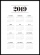Vojta started writing the number of this year 2019202020192020 into the workbook. .. And so he kept going. When he wrote 2020 digits, no longer enjoyed it. How many twos did he write?
• Date game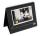Let now is Wednesday. What day is after 248 days? Write the result as a number: 1=Monday, 2=Tuesday, 3=Wednesday, 4=Thursday, 5=Friday, 6=Saturday, 7=Sunday?
• NumbersDetermine the number of all positive integers less than 4183444 if each is divisible by 29, 7, 17. What is its sum?
• MistakeNicol mistake when calculate in school. Instead of add number 20 subtract it. What is the difference between the result and the right result?
• DivideDivide the number 72 in the ratio 7: 2 and calculate the ratio of the numbers found in this order and write down as decimal.
• Unknown numberSamuel wrote unknown number. Then he had add 200000 to the number and the result multiply by three. When it calculated he was surprised, because the result would have received anyway, if write digit to the end of original number. Find unknown number.
• Candy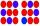How many ways can divide 10 identical candies to 5 children?
• Division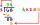Divide by the number 0.2 is the same as multiply by what number?
• FractionsSort fractions ? by its size. Result write as three serial numbers 1,2,3.
• Even / odd numbers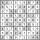a / Using variable n write two consecutive odd numbers. b / the sum of three consecutive odd numbers is 333. What are this numbers?
• ModuloFind x in modulo equation: 47x = 4 (mod 9) Hint - read as: what number 47x divided by 9 (modulo 9) give remainder 4 .
• Unknown xIf we add to unknown number 21, then divide by 6 and then subtract 51, we get back an unknown number. What is this unknown number?
• Decomposition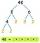Make decomposition using prime numbers of number 155. Result write as prime factors (all, even multiple)
• FractionFraction ? write as fraction a/b, a, b is integers numerator/denominator.
• Year 2020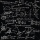The four-digit number divided by 2020 gives a result of 1, **. (Can not be in form 1,*0. ) Write all the options.